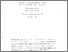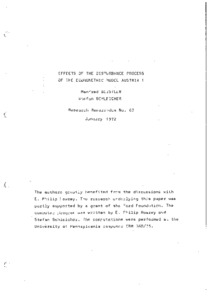# effects of the disturbance process of the econometric model austria 1

Deistler, Manfred and Schleicher, Stefan (January 1972) effects of the disturbance process of the econometric model austria 1. Closed Series > Forschungsberichte / Research Memoranda 63Preview
Text
fo63.pdf

Download (3MB) | Preview

## Abstract or Table of Contents

summary: this paper dealt with an analytical method for calculating the stochastic properties of the econometric model austria 1. using the spectral theory of stationary processes essentially the transformation of the disturbance process by the econometric model was shown. in the time domain these transformations are determined by the coefficients of the model whereas for the frequency domain analysis used in this approach the transfer matrix b(lambda) has been calculated. the effect of this transformation can also be studied by looking at the spectrum matrix of the transformed process. the discussions in this paper were restricted to the probability theoretical aspect of the problem whereas the statistical view was not taken into consideration. although the employed econometric model with 29 equations and lags up to two periods is only of medium size it is probably the largest model upon which this analysis has been applied so far. the reason for this are numerical difficulties whicharise because of the large amount of storage capacity this procedure requires. even with very large computer systems the use of peripherical storage devices for models of this size becomes necessary.;

Item Type: IHS Series Published 26 Sep 2014 10:34 01 Apr 2016 14:07 https://irihs.ihs.ac.at/id/eprint/63

### Actions (login required)View Item